Next: 5.2.9 Parallelization Up: 5.2 Ray Tracing Previous: 5.2.7 Splitting Strategies

To reduce the number of hierarchical traversals within a tree, direct neighbor links to subboxes have been proposed . Apart from single neighbor links between nodes at the same level of the kd-tree, neighbor links trees can be used  to link leaves which correspond to subboxes, directly. A tree is set up for each face of a subbox to enable direct traversals to neighboring subboxes. Hence, using the exit point the next traversed subbox can be obtained by querying the corresponding neighbor links tree.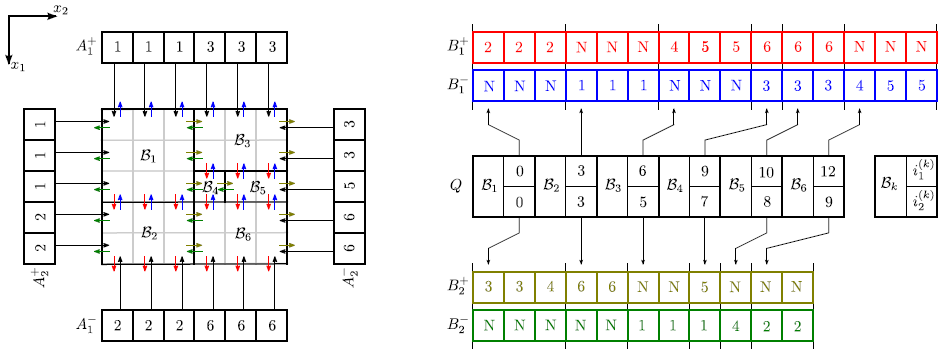In the following discussion a new neighbor links data structure is presented. Using the fact that splitting planes are always aligned with the grid, arrays can be used to store the links to neighbor boxes, as demonstrated for a two-dimensional example in Figure 5.4. The number of links which are required for box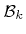, is equal to its surface area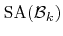(measured in grid spacings).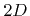different arrays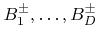hold the neighbor links in a certain grid direction for all boxes. The number of links is equal for opposite directions. Hence, for each boxonly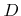array indices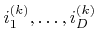have to be stored in order to obtain the corresponding neighbor links. The size of the array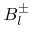is given by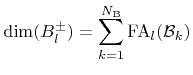(5.25)

where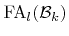is the area of the face for which the normal points in the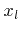-direction: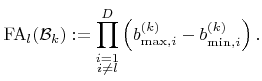(5.26)

Hereand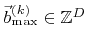are the minimum and maximum index vectors of the corner grid points of box. The index number of the neighboring box at point, where the ray leavesin the positive or negative-direction, is given by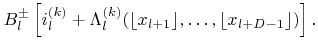(5.27)

Here all subscripts are assumed to be cyclic moduloplus 1 anddenotes the floor function. The bijective function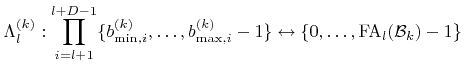(5.28)

is defined as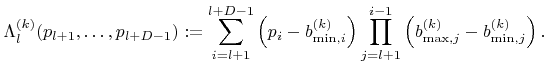(5.29)

The inverse function of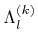is denoted as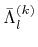.

Additional links from outside of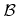allow finding the box which must be traversed first. They are stored in thearrays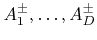with size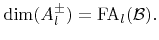(5.30)

The first subbox which is traversed by a ray entering the boxat point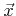in the positive or negative-direction, is given by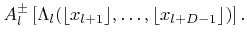(5.31)

The memory requirements of the neighbor links array data structure are primarily given by the total surface area of all boxes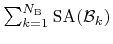. An upper bound estimation can be derived for this sum using (5.23) and the fact that the typical ray traversal costs are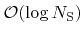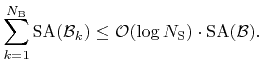(5.32)

For common problems the surface area of the bounding boxscales with the surface size. Therefore, ascaling law can be expected.

Once an appropriate spatial subdivision is found for, the neighbor links data structure can be set up. For subdivisions which are obtained by binary splitting in depth-first order, the neighbor links arrays data structure can be set up in optimal time (directly proportional to the total memory requirements) using Algorithm 5.1.

The neighbor links data structure allows an easy treatment of periodic boundary conditions. The boxes which are situated at the boundary can be directly linked to the corresponding boxes at the opposite boundary.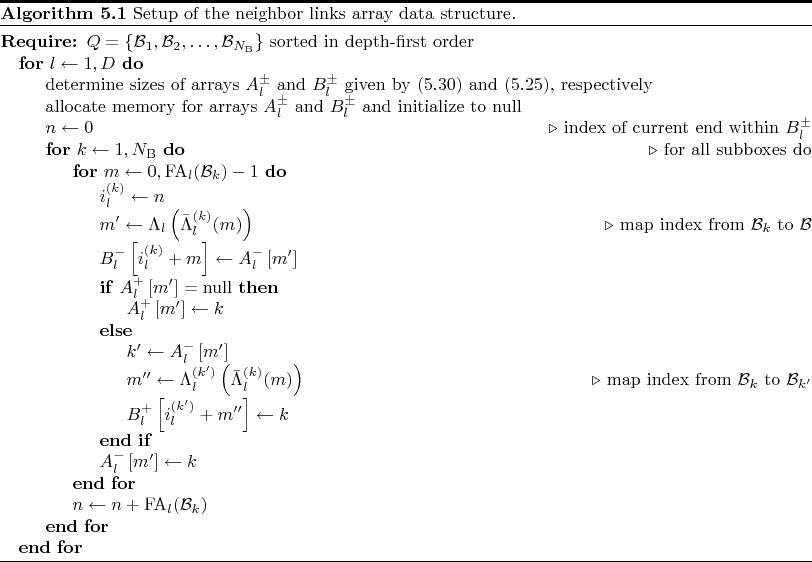Next: 5.2.9 Parallelization Up: 5.2 Ray Tracing Previous: 5.2.7 Splitting Strategies

Otmar Ertl: Numerical Methods for Topography Simulation